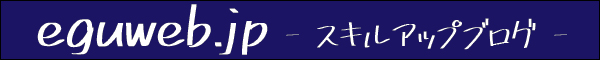# Google Spreadsheet What is the maximum number of rows? Verification of the maximum number of rows

How far can the maximum number of rows in a Google Spreadsheet workbook go? I have verified the following.

So, let’s try to add 1,000,000 rows as a trial.

Yes, … suddenly we have the answer: it seems to be that 5000000 cells is the upper limit!

*Addition: The cell count limit has now been expanded from 5 million to 10 million! The maximum number of cells seems to have been increased from 5 million to 10 million.

## cell count limit

Apparently, there is a “limit of 50000000 cells in a workbook”.

I thought, “Well, it’s 1,000,000 lines, so it should work, right? But here is the point.

If you look closely, the key point is that the “cell count limit is 5,000,000”, not the number of rows.

So, if you have column B~, you cannot try to add row 4,999,999.

## Maximum number of lines

So, it seems that “row x column = 5,000,000 is the maximum” for the maximum number of rows in a spreadsheet.

Finding the square root of 5,000,000, we obtain
= SQRT(5000000)
= 2236.067977

That is, for the same number of matrices
2,236 rows x 2,236 columns (= 4,999,696) is the maximum, and
This means that for the same number of matrices

At 2,236 rows by 2,237 columns, we get.
= 5,001,932, which is
This is over the limit.

Since we have columns from the beginning up to “Z” (column 26), we can use the following formula to calculate the number of rows.
If we keep the initial number of columns and add the number of rows, we get
? ×x 26 = 5,000,000.
The answer to “?” is the upper limit of the number of rows. is the upper limit of the number of rows.

5,000,000 ÷ 26 ＝ 192,307.…

If the number of cells is 27, the number of cells is 5,192,289, which exceeds the upper limit.

I was able to add up to “line 192,307” as expected!

When I try to add row 192,308, I get a warning that “The number of cells in the workbook exceeds the 5000000 limit.” and a warning appears. The result I expected.

## How many lines can we go?

As a test, how many rows can we go if we use only column A?

Cells seem to be able to go up to “exactly” 5,000,000 numbers.

However, if you increase the number of cells on sheet 1 to 5,000,000
You will not be able to add a new sheet.

Since the new sheet has row 1,000 and column Z(26)
When you create a new sheet
You need to leave at least 26,000 cells (1,000 x 26)
cells (1,000 × 26) or more must be left empty in advance.

## If the number of cells is increased too much

Incidentally, if you increase the number of cells too much, the processing will become too heavy and your PC may freeze.

## summary

It would be better to do this when dealing with large numbers of cells or rows, or when you are not working on anything else (-_-;)

Please refer to the following information.en.eguweb.jp